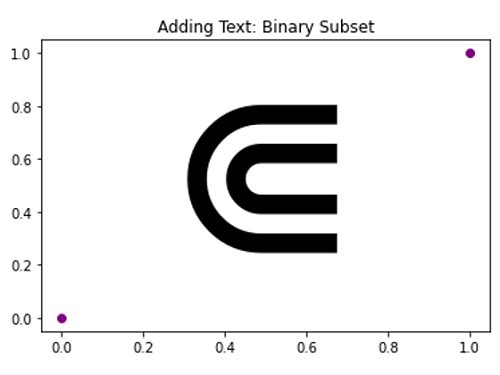# Binary Subset Symbol in Python Plotting

Here, we are going to learn how to add binary subset symbol in Python plotting?
Submitted by Anuj Singh, on September 11, 2020

Binary Subset is a different symbol used in set theory and other mathematical expressions. Python has provided us to use it while plotting.

Following example shows its implementation.

```plt.text(0.25, 0.25, r'\$\Subset\$', fontsize=175)
```

Illustrations:## Python code for binary subset symbol in plotting

```import numpy as np
import matplotlib.pyplot as plt

# Binary Subset
# In text
plt.figure()
plt.plot([0,1],[0,1], 'o', color=  'purple')
plt.text(0.25, 0.25, r'\$\Subset\$', fontsize=175, )
plt.show()

plt.figure()
plt.plot([0,1],[0,1], 'o', color=  'purple')
plt.text(0.25, 0.25, r'\$\Subset\$', fontsize=175, )
plt.axis(False)
plt.show()
```

Output:

```Output is as Figure
```